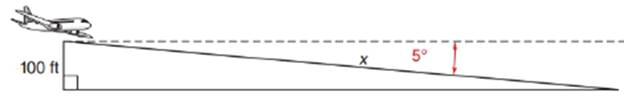Chapter 11.2, Problem 31EElementary Geometry For College St...

7th Edition
Alexander + 2 others
ISBN: 9781337614085

Solutions

Chapter
SectionElementary Geometry For College St...

7th Edition
Alexander + 2 others
ISBN: 9781337614085
Textbook Problem

In Exercise 29 to 37, angle measures should be given to the nearest degree; distances should be given to the nearest tenth of a unit.When an airplane is descending to land, the angle of depression is 5 ∘ . When the plane has a reading of 100 ft. on the altimeter, what is its distance x from touchdown?To determine

To find:

To find the distance x from touchdown by using the following condition,

“When an airplane is descending to land, the angle of depression is 5. When the plane has a reading of 100 ft. on the altimeter”

Explanation

Consider the following figure,

General formula for cosine ratio and sin ratio is given below,

From the given figure the value x ft

Still sussing out bartleby?

Check out a sample textbook solution.

See a sample solution

The Solution to Your Study Problems

Bartleby provides explanations to thousands of textbook problems written by our experts, many with advanced degrees!

Get Started

Under what circumstances is a t statistic used instead of a z-score for a hypothesis test?

Essentials of Statistics for The Behavioral Sciences (MindTap Course List)

In Problems 9-14, evaluate the functions at the given values of the independent variables.

Mathematical Applications for the Management, Life, and Social Sciences

Solve the equations in Exercises 126. (x+1)(x+2)+(x+1)(x+3)=0

Finite Mathematics and Applied Calculus (MindTap Course List)

Let f be the function defined by f(x)={12x2+3ifx12x2+1ifx1 Find f(1), f(0), f(1), and f(2).

Applied Calculus for the Managerial, Life, and Social Sciences: A Brief Approach

Evaluate the indefinite integral. cotxcsc2xdx

Single Variable Calculus: Early Transcendentals, Volume I

True or False: The function y = f(x) graphed at the right could be a solution to y = x + y.

Study Guide for Stewart's Single Variable Calculus: Early Transcendentals, 8th